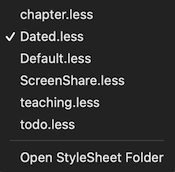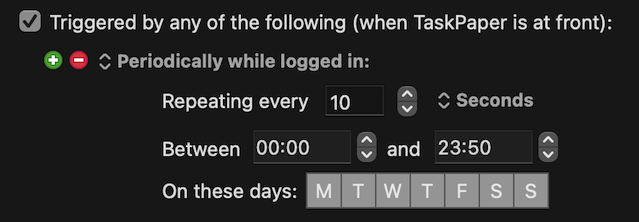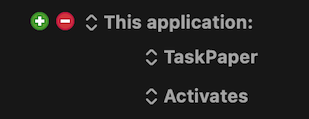# Change task style on due date

Hi, I am new to TaskPaper, just using it a month or two. I have had no problem editing the standard style sheet to get some of the formatting that I want (colors, typeface, etc.).

One thing that I am wonder is whether I can change the style for a task on the due date. I have some tasks that I have tagged @due(date) … I would like them to stand out when that date is reached. Can this been done in the style sheet or do I have to resort to scripting for that?

Thanks.

Yes, but not the way you want I think. You can change styling based on fixed date, but there’s no way to get value for “NOW” to compare that against. You’ll need to use scripting for that.

Here’s one example I found that is doing that:

2 Likes

Also not impossible, I guess, to run a periodic process (perhaps with Hazel, for example) which updates the contents of a .less file

``````// This example colors items red if due on a given date
item[data-due*="2022-03-31"] {
color: rgb(250, 0, 0);
}
``````

2 Likes

Rough sketch, using Keyboard Maestro to periodically trigger an update of a stylesheet called `Dated.less`

`Window > Stylesheet`Every N seconds (or minutes or hours, depending on the macro settings)
(Here – for testing – every 10 seconds, which is probably much too frequent)`Dated.less` is written out afresh at each timed update from two parts:

1. A main set of css styles stored in the macro as `local_taskPaperMainStyle`
2. appended lines which specify some extra styles in relation to dates near today.

The spec for these relative date styles is given in the macro as `local_taskPaperTimedStyles`

and specified, for example, like this:

``````@due[-1, 0, 1] -> color: rgb(250, 0, 0)
@due[2, 3, 4] -> color: rgb(250, 250, 0)
@due[5, 6, 7] -> color: rgb(0, 250, 0)
``````

Which:

1. Start with `@`, naming the tag on which the style will depend
2. Give some integers in JSON list format, where `0` is today, `1` is tomorrow, `-1` is yesterday, etc etc
3. Give, after an `->` arrow, one or more CSS clauses (semi-colon delimited if multiple)

Yielding, for a file in which each item has a different `@due` date, something like this:

where the rules in `local_taskPaperTimedStyles` have been written out at the end of `Dated.less` in CSS format (for today, `2022-03-31`) as something like:

``````item[data-due*="2022-03-30"],
item[data-due*="2022-03-31"],
item[data-due*="2022-04-01"] {
color: rgb(250, 0, 0)
}

item[data-due*="2022-04-02"],
item[data-due*="2022-04-03"],
item[data-due*="2022-04-04"] {
color: rgb(250, 250, 0)
}

item[data-due*="2022-04-05"],
item[data-due*="2022-04-06"],
item[data-due*="2022-04-07"] {
color: rgb(0, 250, 0)
}
``````
Expand disclosure triangle to view JS Source
``````(() => {
"use strict";

// Rob Trew @2022

// main :: IO ()
const main = () => {
const
kme = Application("Keyboard Maestro Engine"),
instanceID = ObjC.unwrap(
\$.NSProcessInfo.processInfo.environment
.objectForKey("KMINSTANCE")
),
kmVar = k => Boolean(instanceID) ? (
kme.getvariable(`local_\${k}`, {
instance: instanceID
})
) : kme.getvariable(k),
fpTPLess = combine(
combine(
applicationSupportPath()
)(
)
)(
kmVar("lessFileName")
),
specLines = lines(
).filter(x => Boolean(x.trim())),

return either(message)(xs => {
const cssDated = xs.join("\n\n");

return either(message)(
() => `Updated \${todayPlus(0)}:\n\${fpTPLess}`
)(
writeFileLR(fpTPLess)(
`\${mainCSS}\n\n\${cssDated}`
)
);
})(
traverseList(datedStyleLR)(specLines)
);
};

// ----------------------- CSS -----------------------

// datedStyleLR :: String -> Either String String
const datedStyleLR = spec => {
// Returns either a message or
// a CSS selector and style
// for a spec like:
// @due[-1,0,1] -> color: rgb(250, 0, 0)
// where 0 signifies today, -1 yesterday,
// 1 tomorrow, etc etc
const
s = spec.trim(),
mbMatches = (
/^@(\w+)(\[[-+ ,0-9]+\])\s*->\s*(.*)\$/gu
).exec(s);

return bindLR(
null === mbMatches ? (
Left(`Could not parse: \${spec}`)
) : jsonParseLR(mbMatches)
)(deltas => {
const
tag = mbMatches,
format = mbMatches,
selectors = deltas.map(
n => `item[data-\${tag}*="\${todayPlus(n)}"]`
)
.join(",\n");

return Right(
`\${selectors} {\n    \${format}\n}`
);
});
};

// ---------------------- DATES ----------------------

// todayPlus :: Int -> IO ISO8601 Day String
const todayPlus = n => {
// A date string for today (+/-) n days
// Today's date where n = 0
// yesterday where n = -1
// tomorrow where n = 1
const dayMilliSeconds = 8.64e+7;

return iso8601Local(
new Date(
(new Date()).getTime() + (
dayMilliSeconds * n
)
)
)
.slice(0, 10);
};

// iso8601Local :: Date -> String
const iso8601Local = dte =>
new Date(dte - (6E4 * dte.getTimezoneOffset()))
.toISOString();

// ----------------------- JXA -----------------------

// alert :: String => String -> IO String
s => {
const sa = Object.assign(
Application("System Events"), {
});

return (
sa.activate(),
sa.displayDialog(s, {
withTitle: title,
buttons: ["OK"],
defaultButton: "OK"
}),
s
);
};

// applicationSupportPath :: () -> String
const applicationSupportPath = () => {
const uw = ObjC.unwrap;

return uw(
uw(\$.NSFileManager.defaultManager
.URLsForDirectoryInDomains(
\$.NSApplicationSupportDirectory,
)
).path
);
};

// writeFileLR :: FilePath ->
// String -> Either String IO FilePath
const writeFileLR = fp =>
// Either a message or the filepath
// to which the string has been written.
s => {
const
e = \$(),
efp = \$(fp).stringByStandardizingPath;

return \$.NSString.alloc.initWithUTF8String(s)
.writeToFileAtomicallyEncodingError(
efp, false,
\$.NSUTF8StringEncoding, e
) ? (
Right(ObjC.unwrap(efp))
) : Left(ObjC.unwrap(
e.localizedDescription
));
};

// --------------------- GENERIC ---------------------

// Left :: a -> Either a b
const Left = x => ({
type: "Either",
Left: x
});

// Right :: b -> Either a b
const Right = x => ({
type: "Either",
Right: x
});

// bindLR (>>=) :: Either a ->
// (a -> Either b) -> Either b
const bindLR = m =>
mf => m.Left ? (
m
) : mf(m.Right);

// combine (</>) :: FilePath -> FilePath -> FilePath
const combine = fp =>
// Two paths combined with a path separator.
// Just the second path if that starts with
// a path separator.
fp1 => Boolean(fp) && Boolean(fp1) ? (
"/" === fp1.slice(0, 1) ? (
fp1
) : "/" === fp.slice(-1) ? (
fp + fp1
) : `\${fp}/\${fp1}`
) : fp + fp1;

// compose (<<<) :: (b -> c) -> (a -> b) -> a -> c
const compose = (...fs) =>
// A function defined by the right-to-left
// composition of all the functions in fs.
fs.reduce(
(f, g) => x => f(g(x)),
x => x
);

// cons :: a -> [a] -> [a]
const cons = x =>
// A list constructed from the item x,
// followed by the existing list xs.
xs => Array.isArray(xs) ? (
[x].concat(xs)
) : "GeneratorFunction" !== xs.constructor.constructor.name ? (
x + xs
) : (
function* () {
yield x;
let nxt = xs.next();

while (!nxt.done) {
yield nxt.value;
nxt = xs.next();
}
}()
);

// either :: (a -> c) -> (b -> c) -> Either a b -> c
const either = fl =>
// Application of the function fl to the
// contents of any Left value in e, or
// the application of fr to its Right value.
fr => e => e.Left ? (
fl(e.Left)
) : fr(e.Right);

// flip :: (a -> b -> c) -> b -> a -> c
const flip = op =>
// The binary function op with
// its arguments reversed.
1 !== op.length ? (
(a, b) => op(b, a)
) : (a => b => op(b)(a));

// fType :: (a -> f b) -> f
const fType = g => {
const s = g.toString();

return s.includes("Right") ? (
Right
) : s.includes("Left") ? (
Left
) : s.includes("Nothing") ? (
Just
) : s.includes("Node") ? (
flip(Node)([])
) : x => [x];
};

// jsonParseLR :: String -> Either String a
const jsonParseLR = s => {
try {
return Right(JSON.parse(s));
} catch (e) {
return Left(
[
e.message,
`(line:\${e.line} col:\${e.column})`
].join("\n")
);
}
};

// liftA2 :: Applicative f => (a -> b -> c) -> f a -> f b -> f c
const liftA2 = f =>
// Lift a binary function to actions.
// liftA2 f a b = fmap f a <*> b
a => b => ({
"(a -> b)": () => liftA2Fn,
"Either": () => liftA2LR,
"Maybe": () => liftA2May,
"Tuple": () => liftA2Tuple,
"Node": () => liftA2Tree,
"List": () => liftA2List,
"Bottom": () => liftA2List
} [typeName(a) || "List"]())(f)(a)(b);

// liftA2LR :: (a -> b -> c) -> Either d a -> Either d b -> Either d c
const liftA2LR = f =>
// The binary function f lifted to a
// function over two Either values.
a => b => bindLR(a)(
x => bindLR(b)(
compose(Right, f(x))
)
);

// lines :: String -> [String]
const lines = s =>
// A list of strings derived from a single string
// which is delimited by \n or by \r\n or \r.
0 < s.length ? (
s.split(/\r\n|\n|\r/u)
) : [];

// pureLR :: a -> Either e a
const pureLR = x =>
// The value x lifted into the Either monad.
Right(x);

// pureT :: String -> f a -> (a -> f a)
const pureT = t =>
// Given a type name string, returns a
// specialised "pure", where
// "pure" lifts a value into a particular functor.
({
"Either": () => pureLR,
"(a -> b)": () => constant,
"Maybe": () => pureMay,
"Node": () => pureTree,
"Tuple": () => pureTuple,
"List": () => pureList
})[t || "List"]();

// traverseList :: (Applicative f) => (a -> f b) ->
// [a] -> f [b]
const traverseList = f =>
// Collected results of mapping each element
// of a structure to an action, and evaluating
// these actions from left to right.
xs => 0 < xs.length ? (() => {
const
vLast = f(xs.slice(-1)),
t = typeName(vLast);

return xs.slice(0, -1).reduceRight(
(ys, x) => liftA2(cons)(f(x))(ys),
liftA2(cons)(vLast)(pureT(t)([]))
);
})() : fType(f)([]);

// typeName :: a -> String
const typeName = v => {
const t = typeof v;

return "object" === t ? (
null !== v ? (
Array.isArray(v) ? (
"List"
) : "Date" === v.constructor.name ? (
"Date"
) : null !== v ? (() => {
const ct = v.type;

return Boolean(ct) ? (
(/Tuple\d+/u).test(ct) ? (
"TupleN"
) : ct
) : "Dict";
})() : "Bottom"
) : "Bottom"
) : {
"boolean": "Bool",
"date": "Date",
"number": "Num",
"string": "String",
"function": "(a -> b)"
} [t] || "Bottom";
};

// showLog :: a -> IO ()
const showLog = (...args) =>
// eslint-disable-next-line no-console
console.log(
args
.map(JSON.stringify)
.join(" -> ")
);

// sj :: a -> String
const sj = (...args) =>
// Abbreviation of showJSON for quick testing.
// Default indent size is two, which can be
// overriden by any integer supplied as the
// first argument of more than one.
JSON.stringify.apply(
null,
1 < args.length && !isNaN(args) ? [
args, null, args
] : [args, null, 2]
);

return (
main()
);
})();
``````
2 Likes

Thanks, any interesting thought. I do have hazel running so maybe i can get something working. I will post here if i do.

Brilliant.

Enjoy it in good health : -)

Incidentally, I think, on reflection, that updating the `.less` file whenever the TaskPaper application is activated may be a better fit than a periodic (timed) event.

In any case, Keyboard Maestro provides a number of different options for the triggering of the update.2 Likes